### IMO Shortlist 2008 problem C2

Kvaliteta:
Avg: 0.0
Težina:
Avg: 6.0
Let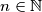$n \in \mathbb N$ and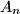$A_n$ set of all permutations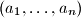$(a_1, \ldots, a_n)$ of the set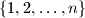$\{1, 2, \ldots , n\}$ for which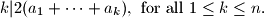Find the number of elements of the set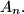$A_n.$
Source: Međunarodna matematička olimpijada, shortlist 2008